# Interpreting medical evidence

Last updated: March 14, 2022

## SummaryCritical appraisal and evidence-based medicine involve the practical application of clinical epidemiology concepts in order to guide clinical decision-making. This requires an evaluation of the quality and applicability of existing research studies to individual clinical scenarios. Appropriate interpretation of the results of a research study in the right context requires a basic understanding of the following foundational concepts (found in the “Epidemiology” article): types of epidemiological studies (e.g., observational studies, experimental studies), common study designs (e.g., case series, cohort studies, case-control studies, randomized controlled trials), causal relationships in research studies, and other reasons for observed associations (e.g., random errors, systematic errors, confounding). This article focuses on an approach to critical appraisal, and epidemiological concepts often encountered in studies of clinical interventions, i.e., measures of association (e.g., relative risk, odds ratios, absolute risk reduction, number needed to treat), measures used to evaluate screening and diagnostic test (e.g., sensitivity, specificity, positive predictive value, negative predictive value), precision, and validity.

The following concepts are discussed separately: measures of disease frequency (e.g., incidence rates, prevalence) commonly used in studies of population health, foundational statistical concepts (e.g., measures of central tendency, measures of dispersion, normal distribution, confidence intervals), and guidance on conducting research projects.

## Evidence-based medicine

• Definition: The practice of medicine in which the physician uses clinical decision-making methods based on the best available current research from peer-reviewed clinical and epidemiological studies with the aim of producing the most favorable outcome for the patient.
• Application in clinical practice
• Define the patient's clinical problem (can be formulated as a PICO question).
• Search for sources of information about the clinical problem.
• Perform a critical appraisal of relevant research studies.
• Apply the information
• Before discussing the research findings with the patient, consider how and to which extent the researched options can improve patient care.
• Present comprehensive, but synthesized evidence to the patient using clear and understandable language.
• Engaged in shared decision-making, considering individual patient's risk profile and preferences.

### Levels of evidence

• Definition: a method used in evidence-based medicine to determine the strength of the findings from a clinical and/or epidemiological study
• Methods: Several different systems exist for assigning levels of evidence.
Levels of evidence 
Level Source of evidence
I
II II.1
• Findings from at least one high-quality, nonrandomized controlled study
II.2
II.3
• Findings from multiple time-series studies or important results from large uncontrolled studies
III
• Expert opinions

A system developed by the US Preventive Task Force (USPSTF) to rate clinical evidence and create guidelines for clinical practice based on medical evidence. 

Grade Net benefit Level of certainty Recommendation
A
• Substantial
• High
• Recommended for patients
B
• Moderate/substantial
• High
• Recommended for patients
C
• Small
• Moderate to high
• Recommended only for certain patients
D
• Zero/negative
• Moderate to high
• Not recommended/discouraged for patients
I
• Cannot be determined
• Low or lacking
• Evidence is insufficient to assess the benefits and harms.
• Might be due to poor quality, conflicting evidence, or complete lack of evidence
• Patients should fully understand the service being offered before accepting it.

Levels of certainty

• High: Further research is unlikely to influence the recommendation.
• Moderate: Further research may influence the recommendation.
• Low: Information is generally insufficient to assess harms and benefits.

## Critical appraisal of research studies

### Applications

• Clinical practice (evidence-based medicine)
• Evaluation of the literature relevant to an individual patient's condition
• Review of updated guidelines on diagnosis and management of medical conditions
• Clinical decision-making
• Gathering background information for a research study
• Serving as a reviewer for a medical journal
• Participation in a journal club

### Procedure

Perform an overall assessment and an in-depth analysis of the different study sections. 

Questions to ask when critically appraising a research paper 
Overall assessment
• Importance
• Is the content relevant to patient care?
• How does this contribute to the existing literature?
• Novelty
• Does the paper evaluate new diagnostic or therapeutic modalities?
• Does the paper evaluate existing diagnostic or therapeutic modalities in a new population or setting?
Title/abstract
• What is the research question?
• Does the abstract appropriately summarize the main methods and results of the paper?
Introduction
• Is the review of the prior literature appropriate/relevant?
• Are the study objectives/aims clearly stated?
• Are relevant hypotheses described?
Methods
• Study design
• What is the study design?
• Is the chosen study design the most appropriate for the research question?
• Participant selection
• What were the study inclusion criteria?
• What were the study exclusion crtieria?
• Are there potential sources of selection bias?
• Study procedures (vary by study type)
• Data collection
• What were the relevant exposures?
• What was the primary outcome?
• Was data collected on potential confounders?
• Data analysis: What statistical tests were used, and were they appropriate?
Results
• Population size
• How many individuals participated in the study?
• What was the response rate (for survey studies)?
• How many participants were lost to follow-up (prospective cohort studies, RCTs)?
• Participant characteristics
• Analysis
• Presentation: Where the results reported according to current guidelines (see Equator network reporting guidelines in “Tips and Links”)?
Discussion
• Did the authors interpret their results in the context of the existing literature?
• Are the study conclusions appropriate based on the findings?
• Is the study generalizable?
• Are the study limitations appropriately addressed?
Other
• Do the study authors have any relevant conflicts of interest?
• Who funded the study?
• Was the study reviewed and approved by an Institutional Review Board?

Reporting guidelines are available for different study types, e.g., CONSORT for randomized trials, STROBE for observational studies, and PRISMA for systematic reviews.

Measures of association can be used to quantify the strength of a relationship between two variables. See also “Measures of disease frequency.”

## Two-by-two table

The degree of association between exposure and disease is typically evaluated using a two-by-two table, which compares the presence/absence of disease with the history of exposure to a risk factor.

Two-by-two table
Disease (outcome)

No disease (no outcome)

Total
Exposure (risk factor) a b a + b
No exposure (no risk factor) c d c + d
Total a + c b + d a + b + c+ d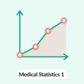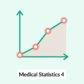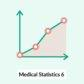## Risk

• Risk factor: a variable or attribute that increases the probability of developing a disease or injury 
• Absolute risk: the likelihood of an event occurring under specific conditions 
• Commonly expressed as a percentage
• Equal to the cumulative incidence, which can be calculated as follows: incidence rate × the time of follow-up
• Aim: to measure the probability of an individual in a study population developing an outcome
• Used in: cohort studies
• Formula: (number of new cases)/(total individuals in a study group) = (a + c)/(a + b + c + d)
• Relative risk: See “Estimates of association strength.”
• Attributable risk: See “Estimates of population impact.”

## Relative risk (RR; risk ratio)

• Description: : the likelihood of an outcome in one group exposed to a potential risk factor compared to the risk in another group that has not been exposed
• Purpose
• To measure how strongly a risk factor is associated with an outcome (e.g., death, injury, disease)
• To help establish disease etiology
• Used in: : cohort studies
• Formula: (incidence of disease in exposed group)/(incidence of disease in unexposed group) = (a/(a + b))/(c/(c + d))
• Interpretation
• RR = 1: Exposure neither increases nor decreases the risk of the defined outcome.
• RR > 1: Exposure increases the risk of the outcome.
• RR < 1: Exposure decreases the risk of the outcome.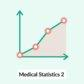## Odds ratio (OR) 

• Description
• Comparison of the odds of an event occurring in one group against the odds of an event occurring in another group
• Odds: the probability of an event occurring divided by the probability of this event not occurring
• Calculated using the two-by-two table
• Purpose: to measure the strength of an association between a risk factor and an outcome
• Used in: : case-control studies
• Formula
• Odds ratio of exposure: compares the odds of exposure among individuals with an outcome (e.g., disease) against the odds of exposure among individuals without an outcome
• Odds of exposure in individuals with disease (i.e., case group): (exposure in individuals with disease)/(no exposure in individuals with disease) = a/c
• Odds of exposure in individuals without disease (i.e., control group): (exposure in individuals without disease)/(no exposure in individuals without disease) = b/d
• Odds ratio: (odds of exposure in individuals with disease)/(odds of exposure in individuals without disease) = (a/c)/(b/d) = ad/bc = (a/b)/(c/d)
• Interpretation
• OR = 1: The outcome is equally likely in exposed and unexposed individuals.
• OR > 1: The outcome is more likely to occur in exposed individuals.
• OR < 1: The outcome is less likely to occur in exposed individuals.
• Rare disease assumption
• Case-control studies do not track participants over time, so they cannot be used to calculate relative risk.
• However, the assumption can be made that if an outcome (e.g., disease prevalence) is rare, the incidence of that outcome is low and the OR is approximately the same as the RR.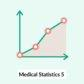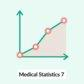## Hazard ratio (HR)

• Description: : a measure of the effect of an intervention on an outcome at any given point in time during the study period 
• Purpose: to help determine how long it takes for an event to occur in individuals in the case group, compared to individuals in the control group
• Used in: survival analysis
• Formula: (observed number of events in exposed group / expected number of deaths in exposed group) at time (t) / (observed number of events in unexposed group/expected number of deaths in unexposed group) at time (t) 
• Interpretation
• HR = 1: no relationship
• HR > 1: The outcome of interest is more likely to occur in exposed individuals.
• HR < 1: The outcome of interest is less likely to occur in exposed individuals.

The RR is the risk of an event occurring by the end of the study period (i.e., cumulative risk), while the HR is the risk of an event occurring at any point in time during the study period (i.e., instantaneous risk). 

The RR, OR, and HR are usually displayed with a corresponding p-value. They are considered statistically significant if the p-value is < 0.05.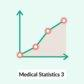## Attributable risk (AR) 

• Description: the absolute difference between the risk of an outcome occurring in exposed individuals and unexposed individuals
• Purpose: to measure the excess risk of an outcome that can be attributed to the exposure
• Used in: cohort studies
• Formulas

## Relative risk reduction (RRR)

• Description: : the proportion of risk in the exposure group after an intervention compared to the risk in the nonexposure group
• Purpose: to determine how much the treatment reduces the risk of negative outcomes
• Used in: cohort studies and cross-sectional studies
• Formulas
• Example: RRR can be used to demonstrate vaccine effectiveness = (risk among unvaccinated – risk among vaccinated)/(risk among unvaccinated) × 100. 

## Absolute risk reduction (ARR; risk difference)

• Description: : the difference between the risk in the exposure group after an intervention and the risk in the nonexposure group (e.g., risk of death)
• Purpose: to show the risk without treatment as well as the risk reduction associated with treatment
• Used in: cohort studies, cross-sectional studies, and clinical trials
• Formula: : (absolute risk in the unexposed group) - (absolute risk in the exposed group) = c/(c + d) – a/(a + b)

## Number needed to treat (NNT)

• Description
• The number of individuals that must be treated, in a particular time period, for one person to benefit from treatment (i.e., to not develop the disease)
• Inversely related to the effectiveness of a treatment
• Purpose: to compare the effectiveness of different treatments
• Used in: clinical trials
• Formula: : 1/ARR

## Number needed to harm (NNH)

• Description
• The number of individuals who need to be exposed to a certain risk factor before one person develops an outcome
• Directly correlates to the safety of the exposure
• Purpose: to determine the potential harms of an intervention
• Used in: clinical trials
• Formula: : 1/AR

## Number needed to screen (NNS)

• Description: the number of individuals who need to be screened in a particular time period in order to prevent one death or adverse event 
• Formula (same as NNT): 1/ARR

## Overview

• Before a diagnostic modality (e.g., laboratory study, imaging study, diagnostic criteria) can be used in clinical practice, it needs to be determined how well the modality can distinguish between individuals with the disease and individuals without the disease.
• A test is compared to the gold standard test using a two-by-two table.
• A two-by-two table can be used to calculate a test's sensitivity, specificity, positive predictive value (PPV), and negative predictive value (NPV).
Features of a two-by-two table summarizing screening or diagnostic test results
Disease No disease Interpretation
Positive test result
• True positive (TP)
• False positive (FP)
• All subjects with positive test results (TP + FP)
• PPV = TP/(TP + FP)
Negative test result
• False negative (FN)
• True negative (TN)
• All subjects with negative test results (FN + TN)
• = TN/(FN + TN)
Interpretation
• All subjects without disease (FP + TN)
• Specificity ( TN/(FP + TN)
• FP/(FP + TN)

### Example 2 x 2 table of a diagnostic test 

Diagnostic test for tuberculosis (TB)
Patients with TB Patients without TB Total
Positive test result 800 (TP) 400 (FP) 1200 (TP + FP)
Negative test result 200 (FN) 3600 (TN) 3800 (FN + TN)
Total 1000 (TP + FN) 4000 (FP + TN) 5000 (TP + FP + FN + TN)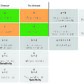## Sensitivity and specificity 

• Every diagnostic test generally involves a tradeoff between sensitivity and specificity.
• Sensitivity and specificity are inversely proportional, meaning that as the sensitivity increases, the specificity decreases, and vice versa.
Overview of sensitivity and specificity of clinical tests
Sensitivity (epidemiology) (true positive rate) Specificity (epidemiology) (true negative rate)
Description
• The proportion of individuals that correctly test positive in a clinical test designed to identify a disease
• The proportion of individuals that correctly test negative in a clinical test designed to identify that disease
Features
• A test with a high sensitivity will yield a rate.
• Tests with high sensitivity are often used for screening purposes.
• If a highly sensitive test yields a negative result, the disease can be ruled out.
• A highly specific test will yield a.
• Tests with high specificity can be used to confirm the diagnosis following a positive screening test.

A highly sensitive test can rule out a disease if negative, and a highly specific test can rule in a disease if positive

## Predictive values 

### Pretest probability

• Description: the probability that a patient with a particular manifestation has a specific disease before the result of the diagnostic test is known
• Features

### Post-test probability

• Description: the probability that a patient has a particular disease after a diagnostic test is carried out
• Features
• Combines disease prevalence and sensitivity and specificity of a test to quantify the likelihood of a patient having a disease
• Can be determined using formulas or nomograms

### Positive predictive value (PPV)

• Description: the proportion of individuals who test positive for a disease that actually have that disease
• Features
• Formula

### Negative predictive value (NPV)

Unlike sensitivity and specificity, which are determined solely by the diagnostic test itself, predictive values are also influenced by disease prevalence.

## Cutoff values

• Definition: dividing points on measuring scales where the test results are divided into different categories
• Positive: has the condition of interest
• Negative: does not have the condition of interest
• Features: Sensitivity, specificity, PPVs, and NPVs vary according to the criterion and/or the cutoff values of the data.
• Interpretation: What happens when a cutoff value is raised or lowered depends on whether the test in question requires a high value (e.g., tumor marker for cancer, lipase for pancreatitis) or a low value (e.g., hyponatremia, agranulocytosis).
• Lowering or raising a cutoff value for a high value test:
• Decreased cutoff value (i.e., broadening the inclusion criteria): lower specificity, higher sensitivity, lower PPV, higher NPV
• Increased cutoff value (i.e., narrowing the inclusion criteria): higher specificity, lower sensitivity, higher PPV, lower NPV
• Lowering or raising a cutoff value for a low value test:
• Decreased cutoff value (i.e., narrowed inclusion criteria): higher specificity, lower sensitivity, higher PPV (decrease in false positives > decrease in true positives), lower NPV (increase in false negatives > increase in true negatives)
• Increased cutoff value (i.e., broadened inclusion criteria): lower specificity, higher sensitivity, lower PPV (increase in true positives > increase in false positives), higher NPV (decrease in false negatives > decrease in true negatives)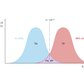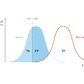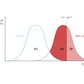## Receiver operating characteristic curve (ROC curve) 

• Description: a graph that compares the sensitivity and specificity of a diagnostic test
• Features
• Shows the tradeoff between clinical sensitivity and specificity for every possible cutoff value, to evaluate the ability of the test to correctly diagnose subjects
• The y-axis represents the sensitivity (i.e., true positive rate) and the x-axis corresponds to 1 - specificity (i.e., the false positive rate).
• A test is considered more accurate the more closely the curve follows the y-axis.
• A test is considered less accurate if the curve is closer to the diagonal.
• The area under the ROC curve (AUROC) also allows the usefulness of tests to be compared: The larger the AUROC, the higher the accuracy of the test. 
• AUROC close to 1.0 indicates that the test has high combined sensitivity and specificity.
• AUROC closer to 0.5 indicates poor discriminative ability.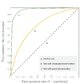## Screening tests

Potential sources of bias in screening tests